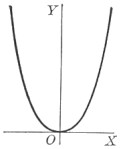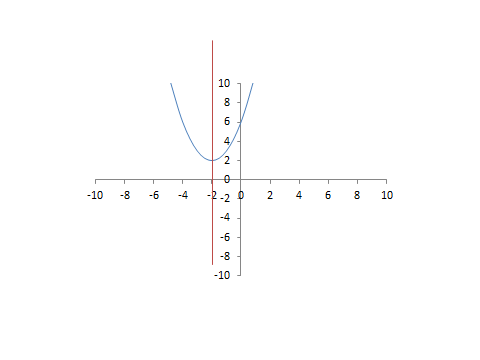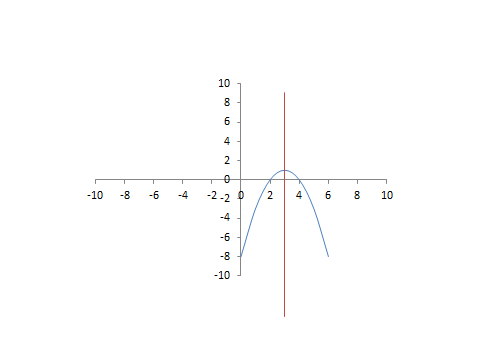# Find the Axis Of Symmetry: Equation, Formula & Vertex

An error occurred trying to load this video.

Try refreshing the page, or contact customer support.

Coming up next: Multiplying Binomials Using FOIL and the Area Method

### You're on a roll. Keep up the good work!

Replay
Your next lesson will play in 10 seconds
• 0:05 Symmetry in a Parabola
• 2:40 Quadratic Form of a Parabola
• 3:20 Axis of Symmetry Equation
• 4:19 The Ordered Pair of the Vertex
• 5:00 Symmetry in a Table of Values
• 5:38 Lesson Summary

Want to watch this again later?

Timeline
Autoplay
Autoplay
Speed

#### Recommended Lessons and Courses for You

Lesson Transcript
Instructor: David Liano
This lesson discusses how to locate the axis of symmetry of a parabola in the standard x-y coordinate plane. Learn how the vertex of the parabola relates to its axis of symmetry and how to determine the axis of symmetry from a quadratic equation.

## The Concept of Symmetry

The word symmetry implies balance. Symmetry can be applied to various contexts and situations. For instance, a marriage could be said to have symmetry if each spouse has an equal share in decision-making when it comes to money matters. But since such matters are not always clear cut, we will confine our discussion today to mathematical contexts.

Symmetry is found in geometry when a figure can be divided into two halves that are exact reflections of each other, as shown in Figure 1. These figures have line symmetry. If we were to fold each figure in half at the red lines (lines of symmetry), the two halves would lie exactly on top of each other.## Axis of Symmetry in a Parabola

In this lesson, our concern is the symmetry of a parabola in the x-y coordinate plane.

Figure 2 shows a parabola that has an axis of symmetry that lies on the y-axis. Notice that the vertex of this parabola is at the ordered pair (0, 0). A parabola's axis of symmetry always goes through the vertex of the parabola. In other words, it is a vertical line that goes through the x-coordinate of the vertex. Therefore, the equation of the axis of symmetry for this parabola is x = 0.As in the geometric figures in Figure 1, if we fold the parabola at the y-axis, the two halves will lie exactly on top of each other. Parabolas always have perfect symmetry. The axis of symmetry of a parabola does not always lie on the y-axis. A parabola can have an axis of symmetry that is left or right of the y-axis, and the parabola can open upward, as in Figure 2, or it can open downward. Parabolas can also be shown as opening up to the left or right, but these types of parabolas are not considered functions and will not be a part of this lesson.

These parabolas each exhibit an axis of symmetry that does not lie on the y-axis. This parabola in Figure 3 has an axis of symmetry that intersects the x-axis at -2. Therefore, the equation of this axis of symmetry is x = -2. The parabola in Figure 4 has an axis of symmetry that intersects the x-axis at 3. Therefore, the equation of this axis of symmetry is x = 3.A parabola is the graph of a quadratic equation. Here is the form of a quadratic equation:Each of the parabolas in Figure 3 and Figure 4 can be expressed as a quadratic equation. The quadratic equation for the parabola in Figure 3 is x^2 + 4x + 6. The quadratic equation for the parabola in Figure 4 is -x^2 + 6x - 8. We can also use these quadratic equations to find the axes of symmetry of the parabolas by applying them to the equation of the axis of symmetry.

## Axis of Symmetry Equation

Here is the equation for the axis of symmetry:Let's look at the quadratic equation for the parabola in Figure 3. The a-value is 1 and the b-value is 4. The equation of the axis of symmetry is:

To unlock this lesson you must be a Study.com Member.

### Register to view this lesson

Are you a student or a teacher?

### Unlock Your Education

#### See for yourself why 30 million people use Study.com

##### Become a Study.com member and start learning now.
Back
What teachers are saying about Study.com

### Earning College Credit

Did you know… We have over 160 college courses that prepare you to earn credit by exam that is accepted by over 1,500 colleges and universities. You can test out of the first two years of college and save thousands off your degree. Anyone can earn credit-by-exam regardless of age or education level.